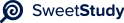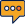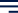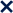•01 / 12100%MODULE FIVE PROBLEM SET
This document is proprietary to Southern New Hampshire University. It and
the problems within may not be posted on any non-SNHU website.
Jacqueline Amoah
1| |
Directions: Type your solutions into this document and be sure to show all steps
for arriving at your solution. Just giving a final number may not receive full credit.
P
ROBLEM
1
Indicate whether the two functions are equal. If the two functions are not equal,
then give an element of the domain on which the two functions have different values.
(a)
f
:
Z
Z
, where
f
(x)
=
x
2
.
g :
Z
Z
, where g(x) =
|
x
|
2
.
(b)
Both
iii
functions
i
are
i
a
i
positive
i
value.x
2
i
is
i
positive
i
and
i
x
i
2
i
can
i
never
i
be
i
negative.
f
:
Z
×
Z
Z
, where
f
(x, y)
=
|
x
+
y
|
.
g :
Z
×
Z
Z
, where g(x, y) =
|
x
|
+
|
y
|
.
These two functions are not equal.
Proof: Let x
=
-2 and y
=
2
f
(x, y)
=
|
2
2
|
=
0
g(x, y) =
|
2
|
+
|
2
2
|
= 2as:
P
ROBLEM
2
The domain and target set of functions f and g is
R
. The functions are defined
f
(x)
=
2x
+
3
g(x) = 5x + 7
(a)
f g?
f (g(x)) = f (5x + 7)
=
2(5x
+
7)
+
3
=
10x
+
14
+
3
f
g
=
10x
+
17
(b)
g
f
?
g(f (x)) = g(2x + 3)
=
5(2x
+
3)
+
7
=
10x
+
15
+
7
g
f =
10x
+
22
(c) (f
g)
1
?
y
=
10x
+
17
x
=
10y
17
Thus
x = (f
g)
1(y)
=
10y
17
(f
g)
1(x)
=
10x
17
(d)
f
1
g
1
?
(f
1
g
1)(x)
=
1(g
1(x))
= f
1(5x 7) = 25x 7 3
= 25x
7
15 = 10x
22
(e) g
1
f
1
?
(g
1
f
1)(x)
=
g
1(f
1)(x))
= g
1(2x
3) = 52x
3
7
= 52x
3
14 = 10x
17Are any of the above equal?
(f
g)
-1
= g
-1
f
-1